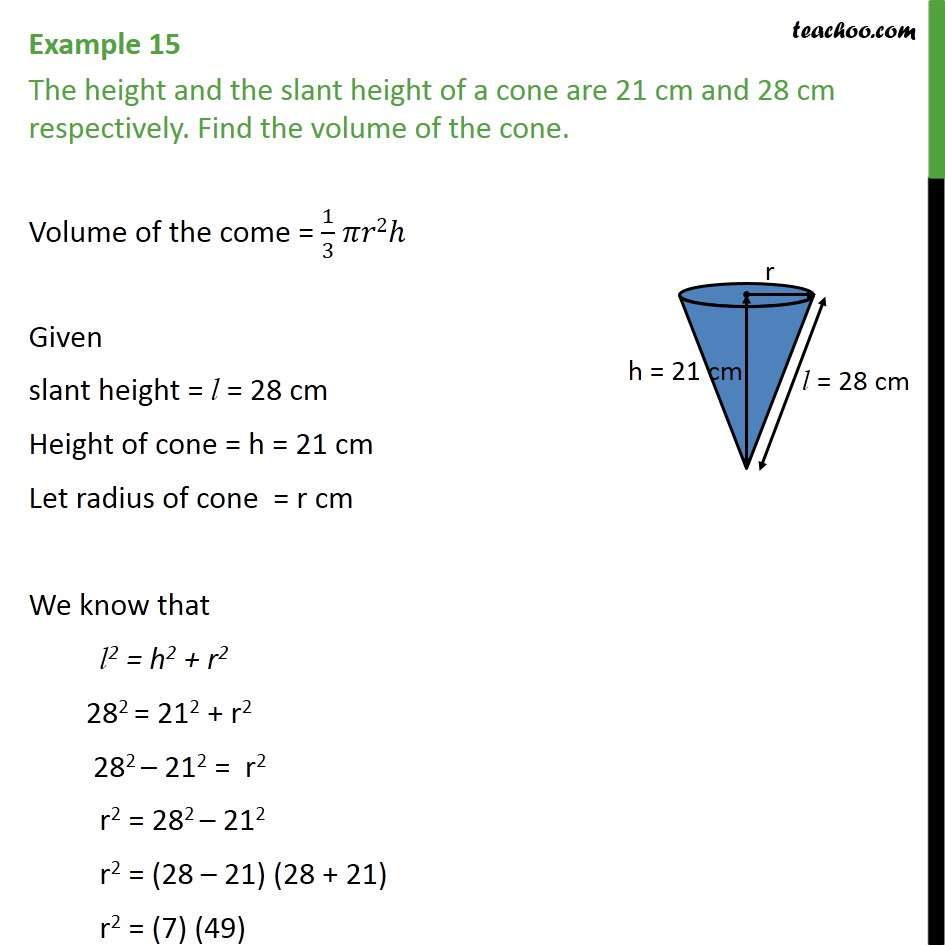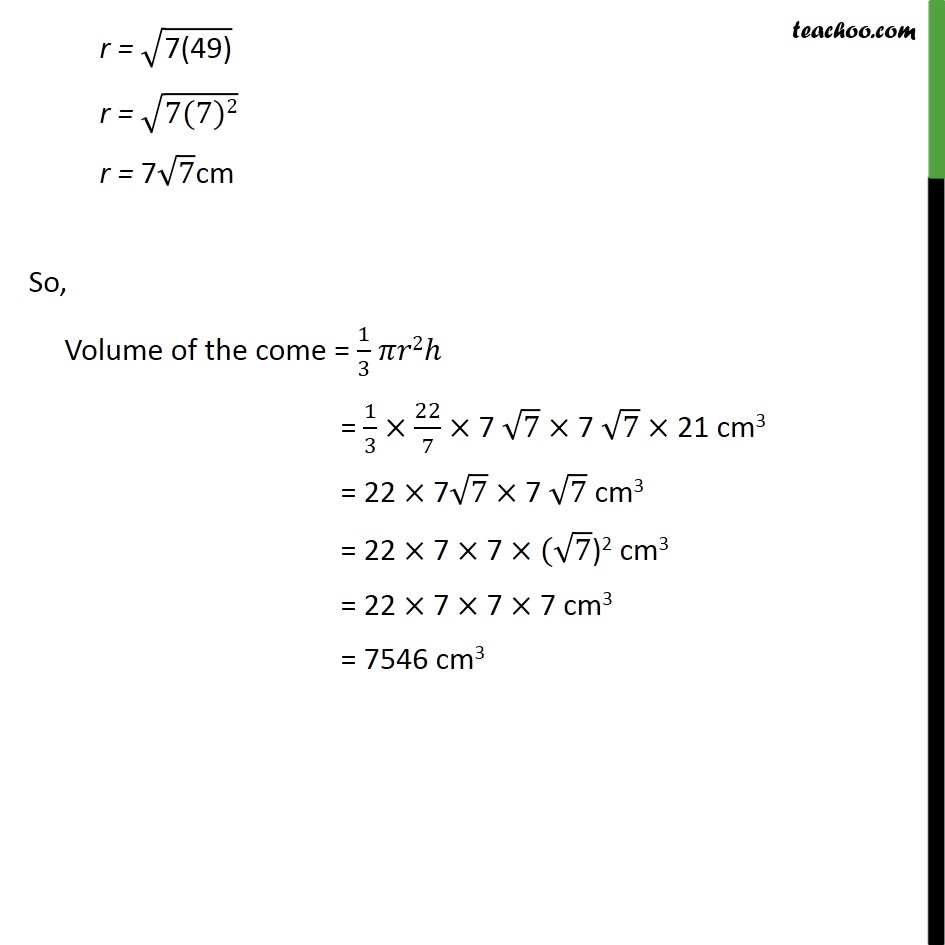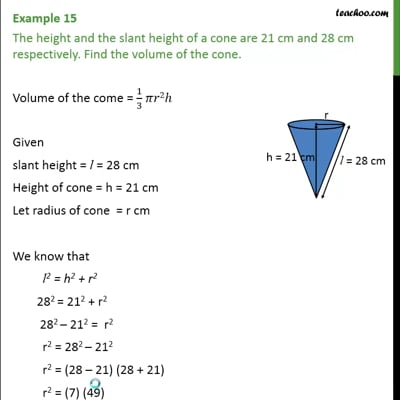Examples

Chapter 13 Class 9 Surface Areas and Volumes (Term 2)
Serial order wiseThis video is only available for Teachoo black users

### Transcript

Example 15 The height and the slant height of a cone are 21 cm and 28 cm respectively. Find the volume of the cone. Volume of the come = 1/3 𝜋𝑟2ℎ Given slant height = l = 28 cm Height of cone = h = 21 cm Let radius of cone = r cm We know that l2 = h2 + r2 282 = 212 + r2 282 – 212 = r2 r2 = 282 – 212 r2 = (28 – 21) (28 + 21) r2 = (7) (49) r = √("7(49)" ) r = √("7(7)2" ) r = 7√7cm So, Volume of the come = 1/3 𝜋𝑟2ℎ = 1/3 × 22/7 × 7 √7 × 7 √7 × 21 cm3 = 22 × 7√7 × 7 √7 cm3 = 22 × 7 × 7 ×(√7)2 cm3 = 22 × 7 × 7 × 7 cm3 = 7546 cm3